﻿ 5. Detailed description of the Actions > 5.16. Time Series > 5.16.2. Smoothen rows (High-Speed action)

# 5.16.2. Smoothen rows (High-Speed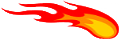action)

Icon: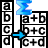Function: SmoothenRows

Property window: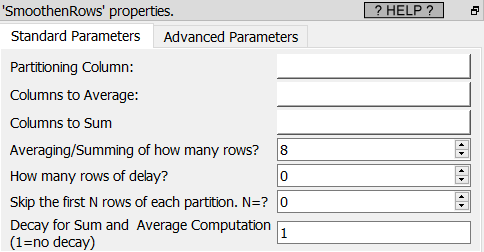Short description:

Smoothen n rows.

Long Description:

This operator is used to create datasets to do time-series prediction.

Let's assume that you have the following original dataset:

+--------------------------------------------------------------+

|                        ORIGINAL TABLE                        |

+--------------------+--------------------+--------------------+

|              ValueA|              ValueB|  TargetToPrediction|

+--------------------+--------------------+--------------------+

| ValueA at time t=1 | ValueB at time t=1 | Target at time t=1 |

| ValueA at time t=2 | ValueB at time t=2 | Target at time t=2 |

| ValueA at time t=3 | ValueB at time t=3 | Target at time t=3 |

| ValueA at time t=4 | ValueB at time t=4 | Target at time t=4 |

| ValueA at time t=5 | ValueB at time t=5 | Target at time t=5 |

|                 ...|                 ...|                 ...|

+--------------------+--------------------+--------------------+

You could directly use the above dataset to create a predictive model that predict the target value at time T in function of the measured value A & B that occurs at the same time T. ...but you won't get any good predictive model because, most of the time, the target value at time T can only be predicted using the A & B values at time T, T-1, T-2, T-3, ...

TIM creates predictive models that,are predicting the Target on a given ROW based on the value contained in the other columns on the SAME ROW, I.e. You need a dataset that contains on each row all the required values to do the prediction for that row.

For example, for time-series prediction, if the target value at time T depends on:

the sum of the A values at time T-2,T-3,T-4

the sum of the B values at time T-2,T-3,T-4

(i.e. the latency of the process to predict is '2' and you must sum '3' different time period), you should have: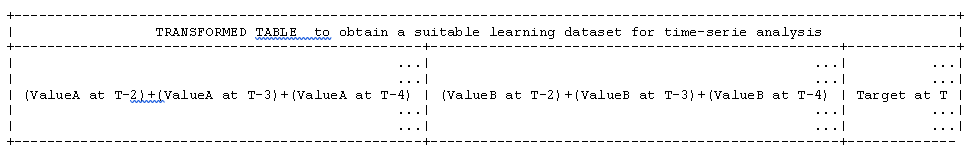The objective of this operator is to obtain the final "TRANSFORMED TABLE" based on the "ORIGINAL TABLE", so that you can easily use TIM to perform a time-series predictive analysis.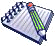NOTE:

You can also obtain the same transformation using the 3 following operators: "Time Travel", "Sum on Columns", "Filter Column".